# Finding the flow of a vector field

docnet
Homework Statement:
Find the flow of the vector field V(x, y) = (y, x)
Relevant Equations:
V(x, y) = (y, x)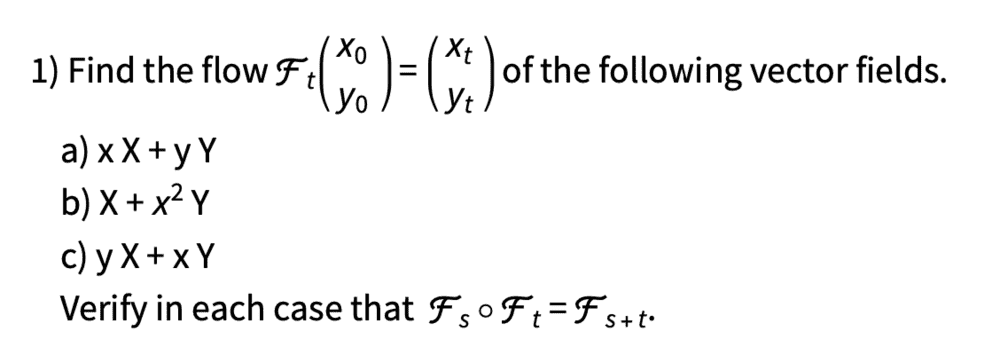In part c, plotting the vector field shows the vector field is symmetric in x and y in the sets {x=y}.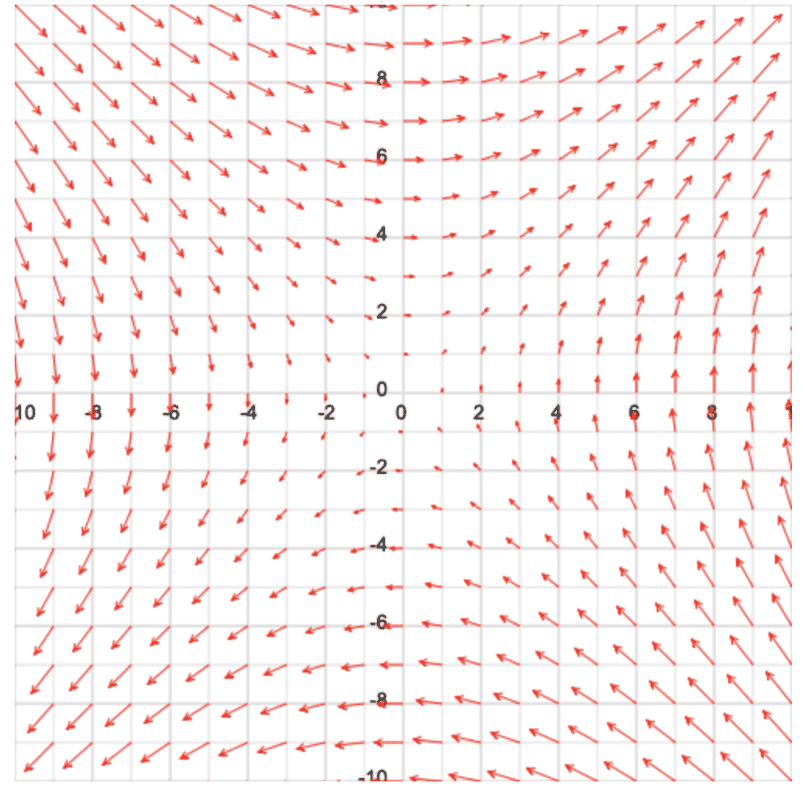in {x=y}, the variables can be interchanged and the solution becomes

x = x°e^t
y = y°e^t

However, these solutions do not work for anywhere except {x=y} and don't satisfy dx/dt = y and dy/dt = x

yX+xY corresponds to the system of ODES

dx/dt = y
dy/dt = x

We eliminate dt from the above system to obtain the following solution to the system of ODES

(x^2)/2 - (y^2)/2=c

However I believe we cannot parametrize this equation to obtain the flow of the vector field.

What I did find from algebraic manipulations is

x = (x°e^t)/2 + (y°e^t)/2 + x°/2 - y°/2
y = (x°e^t)/2 + (y°e^t)/2 - x°/2 + y°/2

which satisfies the values t = 0, x = x°, y= y°

We tried to find the flow by t and s and the calculator tells us this is the wrong answer. Any ideas at all would be appreciated. thank you.

Gold Member
If ##dx/dt=y## and ##dy/dt=x##, then you can substitute one equation into the other to find ##y=dx/dt=d^2y/dt^2## and similarly ##x=dx^2/dt^2.## Then solve and use initial conditions.

docnet
If ##dx/dt=y## and ##dy/dt=x##, then you can substitute one equation into the other to find ##y=dx/dt=d^2y/dt^2## and similarly ##x=dx^2/dt^2.## Then solve and use initial conditions.

Thank you for the advice. We tried this method and obtained the following solutions.

x=x°e^t + c te^t
y=y°e^t + c te^t

We found that the constant c can be set to x°-y° or y°-x° to satisfy dx/dt=y or dy/dt=x. Yet, neither choice satisfies the two initial conditions at the same time.

This is a strange situation because the equations say c = x°-y° and c = y°-x°.

Homework Helper
2022 Award
Thank you for the advice. We tried this method and obtained the following solutions.

x=x°e^t + c te^t
y=y°e^t + c te^t

The solution of $\ddot x = x$ is $$x = A \cosh t + B \sinh t$$ and its derivative is $$\dot x = A\sinh t + B \cosh t.$$ Since $\cosh(0) = 1$ and $\sinh(0) = 0$ it is easy to impose the initial conditions.

We found that the constant c can be set to x°-y° or y°-x° to satisfy dx/dt=y or dy/dt=x. Yet, neither choice satisfies the two initial conditions at the same time.

This is a strange situation because the equations say c = x°-y° and c = y°-x°.

There are two dependent variables Therefore there are two constants of integration. They don't have to be equal.

Staff Emeritus
Homework Helper
yX+xY corresponds to the system of ODES

dx/dt = y
dy/dt = x

We eliminate dt from the above system to obtain the following solution to the system of ODES

(x^2)/2 - (y^2)/2=c

However I believe we cannot parametrize this equation to obtain the flow of the vector field.
You might as well absorb the factor of 1/2 into the constant ##c## so ##x^2-y^2=c##. Depending on the sign of ##c##, you can parameterize using the hyperbolic functions. If ##c>0##, you can write
\begin{align*}
x &= \sqrt{c} \cosh (t-t_0) \\
y &= \sqrt{c} \sinh (t-t_0)
\end{align*} If ##c<0##, you'd need to swap ##\cosh## and ##\sinh## and stick a minus sign in the square root.

Note this is the same solution as the one @pasmith gives in post #4.

What I did find from algebraic manipulations is

x = (x°e^t)/2 + (y°e^t)/2 + x°/2 - y°/2
y = (x°e^t)/2 + (y°e^t)/2 - x°/2 + y°/2

which satisfies the values t = 0, x = x°, y= y°
You seem to be losing solutions along the way as there should be ##e^{-t}## terms as well. I'm guessing when you took a square root somewhere, you neglected the negative solution.

docnet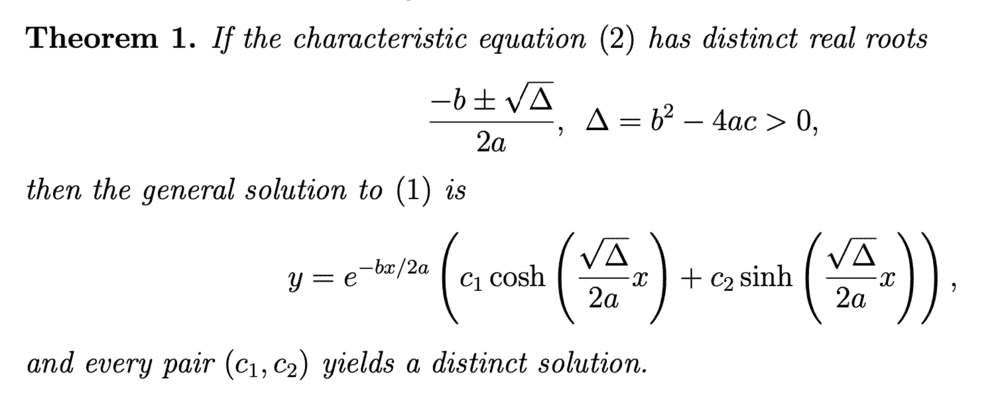Thank you Pasmith and vela, you are right, this method solves the problem!

using the hyperbolic solution to second order odes, I found the solution

x= x cosh t + y sinh t
y= x sinh t + y cosh t

here is a graph of the flow when x = -1 and y = -2 parametrized by t.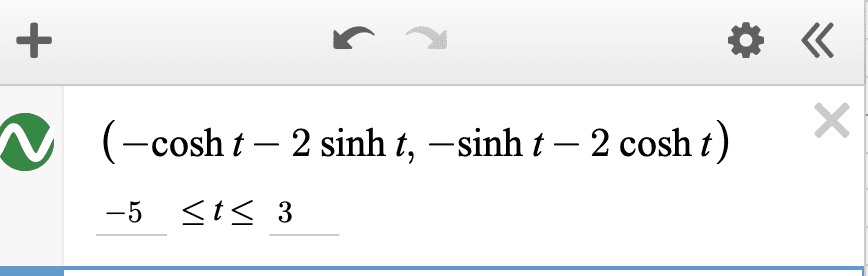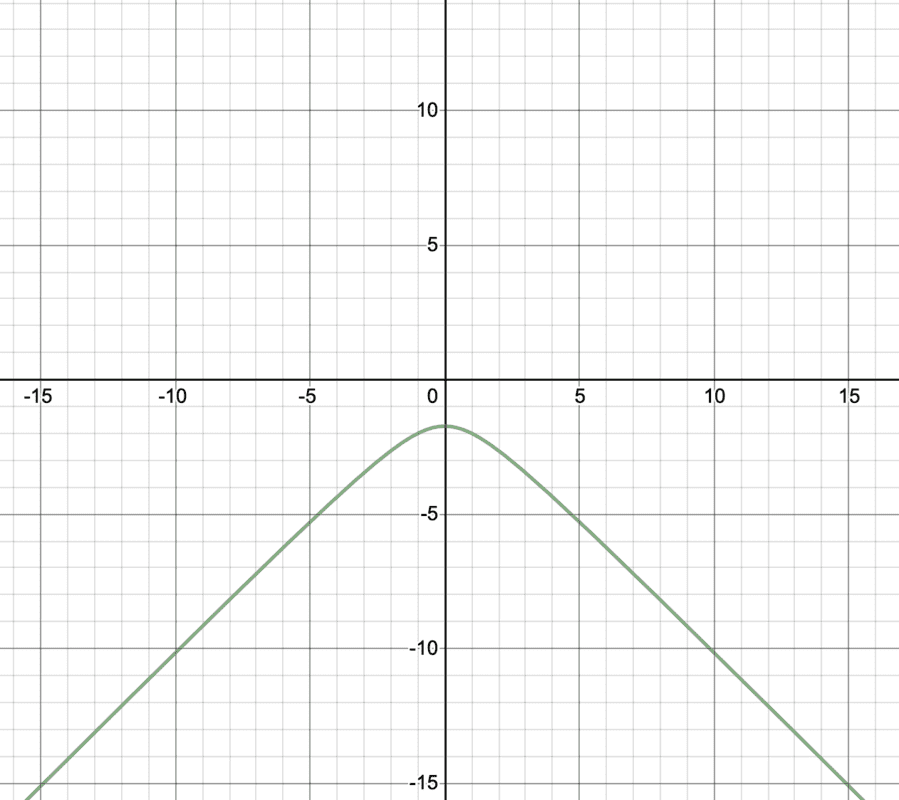vela, you are right, there are e^-t terms that I missed as well. I was tired after trying for many hours to solve this problem.

Last edited: